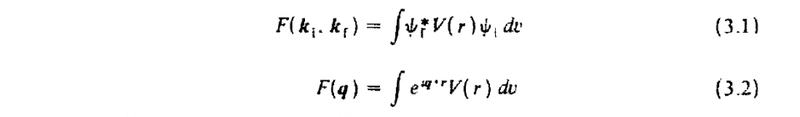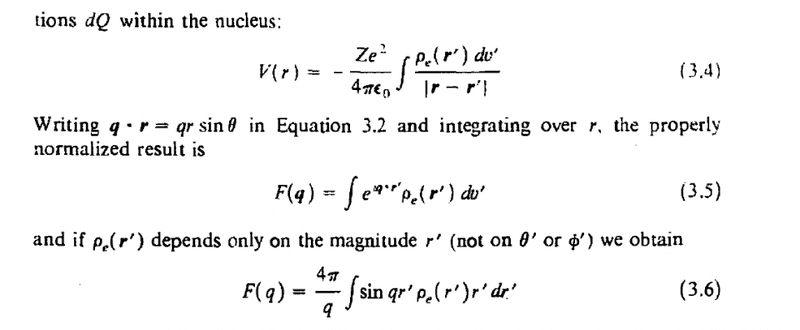# How did this integral come into being?

• I
Hi, this is actually from a text of Krane's Introductory Nuclear Physics.
I don't really understand how did he arrive (3.5) from (3.2) and (3.4), and the reason for setting q ⋅ r = qrsinθ is also confusing.

This is the first time I post and I'm not really sure whether this is the correct section for this kind of question, so if it's the wrong place to post please just tell me. Thanks!blue_leaf77
Homework Helper
Substitute (3.4) into (3.2) to obtain
$$F(\mathbf q) \propto \int e^{i\mathbf q \cdot \mathbf r} \int \frac{\rho(\mathbf r')}{|\mathbf r - \mathbf r'|} dv' dv = \int \rho(\mathbf r') \left( \int \frac{e^{i\mathbf q \cdot \mathbf r}}{|\mathbf r - \mathbf r'|} dv \right)dv'$$
Compute the integral in the parentheses first by a change of variable ##\mathbf u = \mathbf r - \mathbf r'##,
$$\int \frac{e^{i\mathbf q \cdot \mathbf r}}{|\mathbf r - \mathbf r'|} dv = e^{i\mathbf q \cdot \mathbf r'} \int \frac{e^{i\mathbf q \cdot \mathbf u}}{u} dv$$
where now ##dv = u^2 \sin \theta du\,d\theta\,d\phi##.Here ##\theta## is the angle between ##\mathbf q## and ##\mathbf u##, thus ##\mathbf q \cdot \mathbf u = qu \cos \theta## (I think it should be cosine instead of sine as your source states). The integral above becomes
$$e^{i\mathbf q \cdot \mathbf r'} \int e^{iqu \cos \theta} u \sin \theta du\,d\theta\,d\phi = -e^{i\mathbf q \cdot \mathbf r'} \int_0^\infty \int_0^\pi \int_0^{2\pi} e^{iqu \cos \theta} u du\,d\cos\theta\,d\phi$$
Now solve this last integral.
Hint: Solve the integral over ##\theta## first. The integral over ##\phi## is trivial. As for the remaining integral over ##u##, employ a limiting condition by multiplying the integrand with a function of the form ##e^{-bu}##, then apply the limit ##b\to 0## after calculating the integral.

•Homework Helper
Gold Member
Question I have-(I tried working this earlier and got something similar to @blue_leaf77 calculation above), but I got that there should be a ## q^2 ## in the denominator of equation (3.5) which will be a ## q^3 ## in the denominator of equation (3.6). Did I compute it incorrectly? I'm double-checking my algebra. I'm hoping the author has (3.5) and (3.6) correct, but I'm not so sure. (If you compute blue_leaf77's last integral with the substitution ## u'=qu ##, you get a ## q^2 ## in the denominator.)

Last edited:
blue_leaf77
Homework Helper
Question I have-(I tried working this earlier and got something similar to @blue_leaf77 calculation above), but I got that there should be a ## q^2 ## in the denominator of equation (3.5) which will be a ## q^3 ## in the denominator of equation (3.6). Did I compute it incorrectly? I'm double-checking my algebra. I'm hoping the author has (3.5) and (3.6) correct, but I'm not so sure. (If you compute blue_leaf77's last integral with the substitution ## u'=qu ##, you get a ## q^2 ## in the denominator.)
Yes you are right I think there should be ##1/q^2## in equation (3.5).

Sorry to resurrect this thread, but I'm not getting it. Like y'all, I have
$$e^{i\mathbf q \cdot \mathbf r'} \int e^{iqu \cos \theta} u \sin \theta du\,d\theta\,d\phi$$
When iterated (and doing the ##\phi## part)
$$2 \pi e^{i\mathbf q \cdot \mathbf r'} \int_0^\infty u \int_{-1}^1 e^{iqu \cos \theta} d\cos \theta \, du$$
Solving the inner integral
$$2 \pi e^{i\mathbf q \cdot \mathbf r'} \int_0^\infty u \frac{e^{iqu} - e^{-iqu}}{i q u} du = \frac{2 \pi}{q} e^{i\mathbf q \cdot \mathbf r'} \int_0^\infty \sin(q u) du$$
But this final expression does not appear to converge. Does Krane mean that the integration should take place only up to ##r'##?

A similar conversation about the nuclear form factor appears here (pg 5): http://www.umich.edu/~ners311/CourseLibrary/bookchapter10.pdf
but he simplifies the expression by eliminating the exponential based on a simplifying assumption that the projectile is unaffected by the potential (???). Another source (http://hitoshi.berkeley.edu/221b/scattering2.pdf equation 16) gets an identical result to Krane.

Last edited:
Homework Helper
Gold Member
On this last integral, evaluate as @blue_leaf77 suggested as ## I=\int\limits_{0}^{+\infty} \sin(qx) \,dx=\frac{1}{q} \int\limits_{0}^{+\infty} \sin(x) \, dx=\frac{1}{q} \int\limits_{0}^{+\infty} e^{-bx} \sin(x) \, dx ## and then take the limit as ## b \rightarrow 0 ##. I believe the result is ## I=\frac{1}{q} ##. (You get a factor of ## \frac{1}{b^2+1} ## in evaluating the integral).## \\ ## Incidentally, the textbook left off the factor ## Ze^2/\epsilon_o ## in the final result, and it should be a ## q^3 ## in the denominator of equation (3.6) as previously mentioned in post #3. ## \\ ## Also, on your last equation in post #6, the ## 2 \pi ## on the left becomes a ## 4 \pi ## on the right. (There's a factor of ## 2i ## in the denominator of the complex expression for ## \sin{x} ##).

Last edited:
blue_leaf77
Homework Helper
Incidentally, the textbook left off the factor Ze2/ϵoZe2/ϵo Ze^2/\epsilon_o in the final result, and it should be a q3q3 q^3 in the denominator of equation (3.6) as previously mentioned in post #3. \\
You are right, without knowing the full text it's difficult to tell whether these are mistakes or that the author has said something about the definition in each quantity. Furthermore, the right hand sides of (3.1) and (3.2) have different units.

•Homework Helper
Gold Member
A similar conversation about the nuclear form factor appears here (pg 5): http://www.umich.edu/~ners311/CourseLibrary/bookchapter10.pdf
but he simplifies the expression by eliminating the exponential based on a simplifying assumption that the projectile is unaffected by the potential (???). Another source (http://hitoshi.berkeley.edu/221b/scattering2.pdf equation 16) gets an identical result to Krane.
Additional comment: The Berkeley paper looks like the better of the two, and see equation (15) in the Berkeley paper along with equation (16). They do get the extra ## q^2 ## in the denominator as I have previously mentioned.

•RGann and blue_leaf77
I think this is the answer. Strangely, the text seems to confuse the scattering amplitude with the form factor. As Charles says, the extra ##1/q^2## is factored out of the form factor. This is also present in F. Scheck, Electroweak and Strong Interactions, eqns 2.5-2.6. Only Krane seems to skip over this step. Thanks all.

•